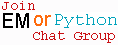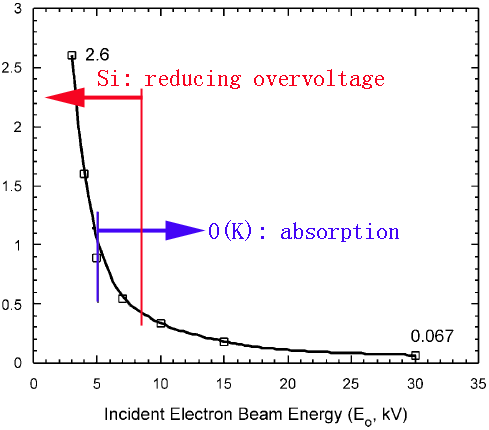X-Ray Intensity Affected by Overvoltage
- Practical Electron Microscopy and Database -
- An Online Book -Microanalysis | EM Book                                                                   http://www.globalsino.com/EM/

Figure 4594a shows EDS analysis of materials consisting of Al, Pt, and O elements. At high accelerating voltage (30 kV), the spectrum shows a dominant Pt(M) (~2.1 keV) peak. The intensity of O(K) (~0.5 keV) X-rays is very weak due to the matrix absorption. As the voltage is reduced to middle voltage and then to low voltage (4 keV), the proportion of Pt measured in the Pt(M) (~2.1 keV) X-ray lines declines and the Pt(L) (~8.2 keV, not shown) lines disappear in the middle voltage range, due to a vanishing overvoltage U, and at low voltages (e.g. 4 keV) an O(K) (~0.5 keV) signal appears and increases in strength, due to reaching the maximum ionization cross section at optimum U.Figure 4594a. EDS analysis of materials consisting of Al, Pt, and O elements .

Figure 4594b shows the ratio of O and Si X-rays signals, taken from quartz (SiO2), as a function of incident electron beam energy (E0), detected with a Si(Li) ATW detector. The plot extends from a very low O/Si ratio (0.067) at 30 kV through an analysis at 5 kV which closely reflects the correct chemistry of the sample to analyses at low voltages of < 5 kV with O/Si >> 1. The final data point is 2.6 at 2.7 kV. Above 5 kV the accuracy of the analyses are limited primarily by differential absorption of the O(K) X-ray signal. At low voltage the under representation of Si reflects the reduction in the fluorescent yield with reducing overvoltage (U).Figure 4594b. The ratio of O and Si X-rays signals, taken from quartz (SiO2), as a function of incident electron
beam energy (E0), detected with a Si(Li) ATW detector .

 Edward D. Boyes, Analytical potential of EDS at low voltages, Mikrochim. Acta 138, 225-234 (2002).

 ;